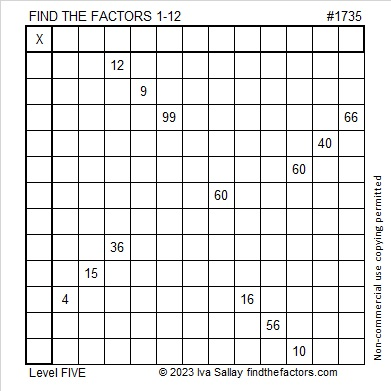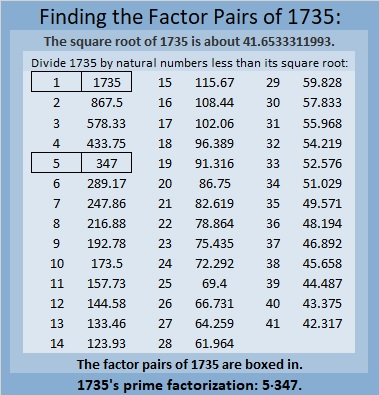# 1735 What Amazing Mathematical Pattern I Noticed Today!

Contents

### Today’s Puzzle:

Will the common factor of 60 and 10 be 5 or will it be 10? Don’t guess. There is a better place to start this puzzle. After using logic to find some of the other factors, you will know if you should use 5 or 10. Don’t let me trip you up!### Factors of 1735:

• 1735 is a composite number.
• Prime factorization: 1735 = 5 × 347.
• 1735 has no exponents greater than 1 in its prime factorization, so √1735 cannot be simplified.
• The exponents in the prime factorization are 1 and 1. Adding one to each exponent and multiplying we get (1 + 1)(1 + 1) = 2 × 2 = 4. Therefore 1735 has exactly 4 factors.
• The factors of 1735 are outlined with their factor pair partners in the graphic below.### More About the Number 1735 and an Amazing Pattern:

1735 is the hypotenuse of a Pythagorean triple:
1041-1388-1735, which is (3-4-5) times 347.

1735 is 567 in base 18 because
5(18²) + 6(18) + 7(1) = 1735.

I know neither of those facts is amazing, but read on:

1735 is the sum of two, five, and ten consecutive positive numbers:
867 + 868 = 1735,
345 + 346 + 347 + 348 + 349 = 1735, and
169 + 170 + 171 + 172 + 173 + 174 + 175 + 176 + 177 + 178 = 1735.

1735 is the difference of two squares in two different ways:
868² – 867² = 1735, and
176² – 171² = 1735.

That first difference of two squares used the two consecutive numbers I listed above in red. Since mathematics is the study of patterns, I wondered if there would also be a relationship of squares with the ten consecutive numbers in blue. I typed into my calculator:
178² – 177² + 176² – 175² + 174² – 173² + 172² – 171² + 170² – 169² =

Guess what the answer was? If you guessed 1735, you would be right! Oh, mathematics is certainly filled with amazing patterns! I’m thrilled that I spotted this one! It appears something similar can be written whenever a number is the sum of an even amount of consecutive numbers! I find that amazing!

I also noticed that the second difference of two squares noted above is contained in the string. I underlined those two squares. Since the difference of those squares by themselves equals the whole string, it follows that the rest of those squares added and subtracted equal zero. That means the sum of the rest of the positive terms must equal the sum of the rest of the negative terms:
178² + 174² + 172² + 170² = 120144 = 177² + 175² + 173² + 169².
I’ve never found a string of four squares equal to another string of four squares before, so naturally, I’m excited! I’m anxious to apply this pattern to other numbers that are the sum of ten consecutive numbers. For example, the first ten numbers add up to 55.
10² – 9² + – 7² + 6² – 5² + 4² – 3² + 2² – 1² =55. And since 8² – 3² = 55, it follows that
10²  + 6² + 4² + 2² = 156 = 9² + 7² + 5²+ 1².
It is no longer “impossible” for me to find these equal sums! Here’s another that connects this year with other recent years:We can all hope this equation doesn’t predict the future. Ha ha! I’m confident it is NOT a bad omen!

This site uses Akismet to reduce spam. Learn how your comment data is processed.